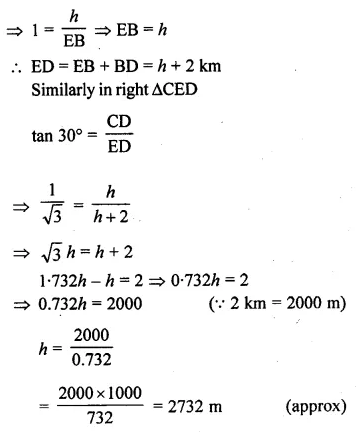# ML Aggarwal Class 10 Solutions for ICSE Maths Chapter 20 Heights and Distances Ex 20

## ML Aggarwal Class 10 Solutions for ICSE Maths Chapter 20 Heights and Distances Ex 20

ML Aggarwal Class 10 Solutions for ICSE Maths Chapter 20 Heights and Distances Ex 20

Question 1.
An electric pole is 10 metres high. If its shadow is 10√3 metres in length, find the elevation of the sun.
Solution:Question 2.
The angle of elevation of the top of a tower from a point on the ground and at a distance of 150 m from its foot is 30°. Find the height of the tower correct to one place of decimal
Solution:Question 3.
A ladder is placed against a wall such that it just reaches the top of the wall. The foot of the ladder is 1.5 metres away from the wall and the ladder is inclined at an angle of 60° with the ground. Find the height of the wall.
Solution:Question 4.
What is the angle of elevation of the sun when the length of the shadow of a vertical pole is equal to its height?
Solution:Question 5.
A river is 60 m wide. A tree of unknown height is on one bank. The angle of elevation of the top of the tree from the point exactly opposite to the foot of the tree on the other bank is 30°. Find the height of the tree.
Solution: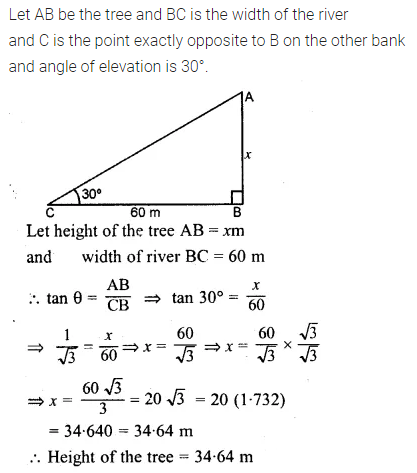Question 6.
From a point P on level ground, the angle of elevation of the top of a tower is 30°. If the tower is 100 m high, how far is P from the foot of the tower?
Solution:Question 7.
From the top of a cliff 92 m high, the angle of depression of a buoy is 20°. Calculate to the nearest metre, the distance of the buoy from the foot of the cliff. (2005)
Solution:Question 8.
A boy is flying a kite with a string of length 100 m. If the string is tight and the angle of elevation of the kite is 26°32′, find the height of the kite correct to one decimal place, (ignore the height of the boy).
Solution:Question 9.
An electric pole is 10 m high A steel wire tied to the top of the pole is affixed at a point on the ground to keep the pole upright. If the wire makes an angle of 45° with the horizontal through the foot of the pole, find the length of the wire.
Solution: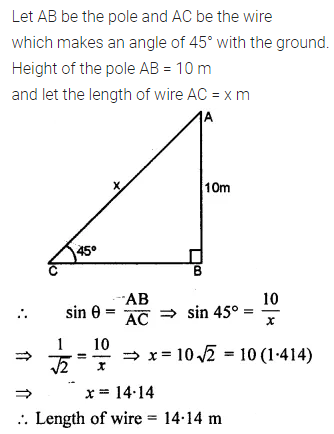Question 10.
A bridge across a river makes an angle of 45° with the river bank. If the length of the bridge across the river is 200 metres, what is the breadth of the river?Solution: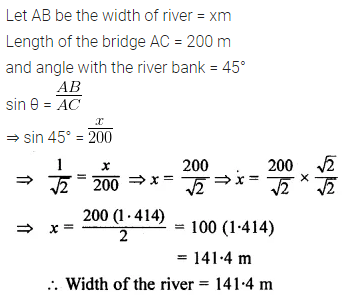Question 11.
A vertical tower is 20 m high. A man standing at some distance from the tower knows that the cosine of the angle of elevation of the top of the tower is 0.53. How far is he standing from the foot of the tower? (2001)
Solution: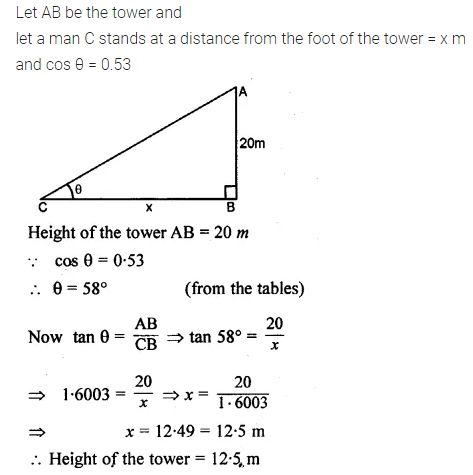Question 12.
The upper part of a tree broken by the wind, falls to the ground without being detached. The top of the broken part touches the ground at an angle of 38°30′ at a point 6 m from the foot of the tree. Calculate.
(i) the height at which the tree is broken.
(ii) the original height of the tree correct to two decimal places.
Solution: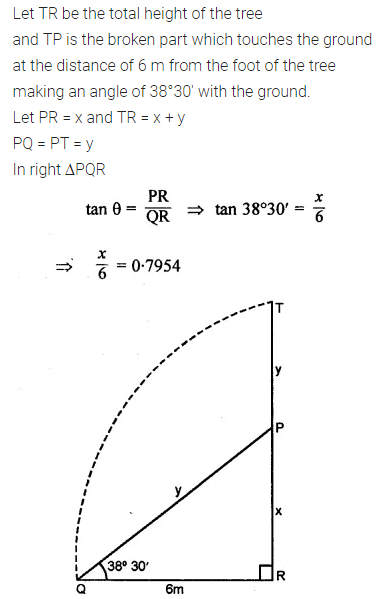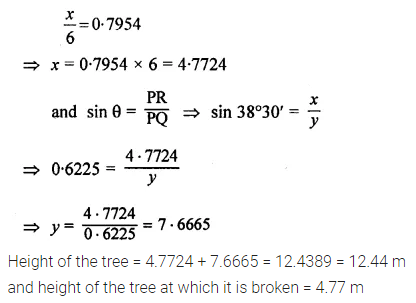Question 13.
An observer 1.5 m tall is 20.5 metres away from a tower 22 metres high. Determine the angle of elevation of the top of the tower from the eye of the observer.
Solution:Question 14.
From a boat 300 metres away from a vertical cliff, the angles of elevation of the top and the foot of a vertical concrete pillar at the edge of the cliff are 55°40′ and 54°20′ respectively. Find the height of the pillar correct to the nearest metre.Solution:Question 15.
From a point P on the ground, the angle of elevation of the top of a 10 m tall building and a helicopter hovering over the top of the building are 30° and 60° respectively. Find the height of the helicopter above the ground.
Solution: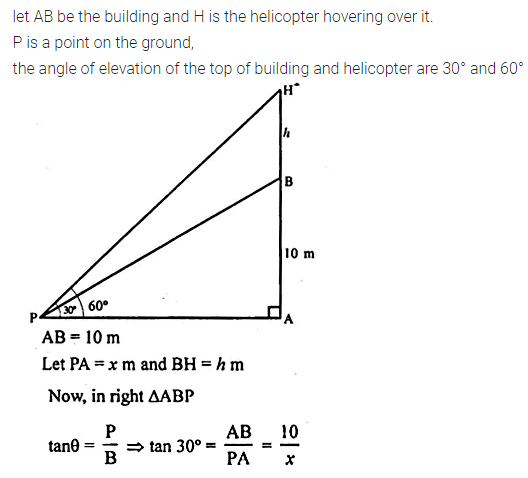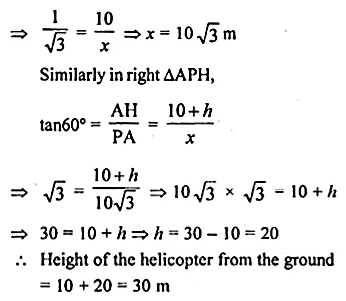Question 16.
An aeroplane when flying at a height of 3125 m from the ground passes vertically below another plane at an instant when the angles of elevation of the two planes from the same point on the ground are 30° and 60° respectively. Find the distance between the two planes at the instant.
Solution: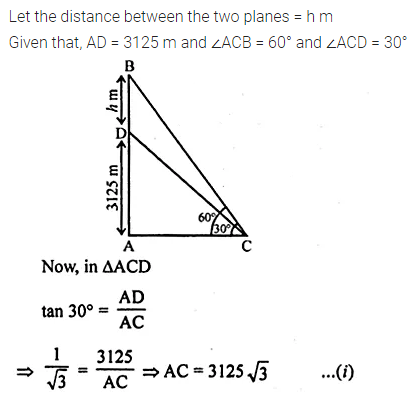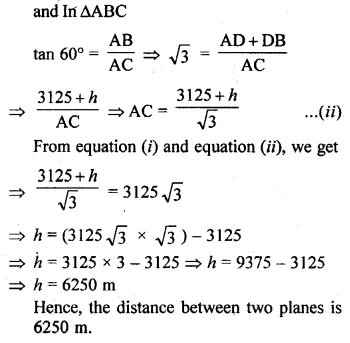Question 17.
A person standing on the bank of a river observes that the angle subtended by a tree on the opposite bank is 60° ; when he retires 20 m from the bank, he finds the angle to be 30°. Find the height of the tree and the breadth of the river. .
Solution: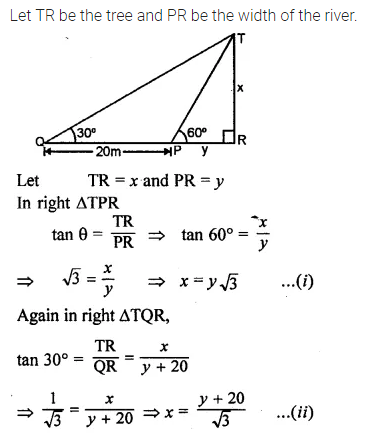Question 18.
The shadow of a vertical tower on a level ground increases by 10 m when the altitude of the sun changes from 45° to 30°. Find the height of the tower, correct to two decimal places. (2006)
Solution:Question 19.
From the top of a hill, the angles of depression of two consecutive kilometre stones, due east are found to be 30° and 45° respectively. Find the distance of two stones from the foot of the hill.
Solution: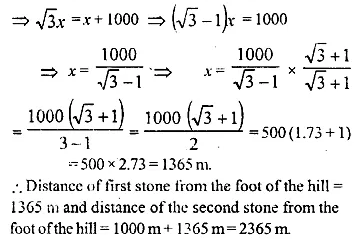Question 20.
A man observes the angles of elevation of the top of a building to be 30°. He walks towards it in a horizontal line through its base. On covering 60 m the angle of elevation changes to 60°. Find the height of the building correct to the nearest me he.
Solution:Question 21.
At a point on level ground, the angle,of elevation of a vertical lower is found to be such that its tangent is $$\\ \frac { 5 }{ 12 }$$. On walking 192 m towards the tower,the tangent of the angle is found to be $$\\ \frac { 3 }{ 4 }$$. Find the height of the tower. (1990)
Solution: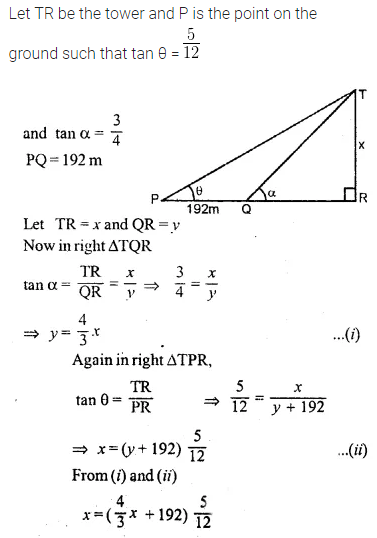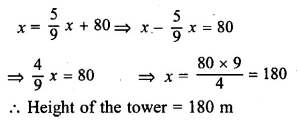Question 22.
In the figure, not drawn to scale, TF is a tower. The elevation of T from A is x° where tan x = $$\\ \frac { 2 }{ 5 }$$ and AF = 200 m. The elevation of T from B, where AB = 80 m, is y°. Calculate :
(i) The height of the tower TF.
(ii) The angle y, correct to the nearest degree. (1997)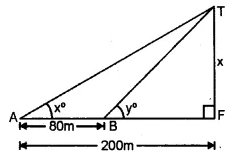Solution:Question 23.
From the top of a church spire 96 m high, the angles of depression of two vehicles on a road, at the same level as the base of the spire and on the same side of it are x° and y°, where tan x° = $$\\ \frac { 1 }{ 4 }$$ and tan y° = $$\\ \frac { 1 }{ 7 }$$. Calculate the distance between the vehicles. (1994)
Solution: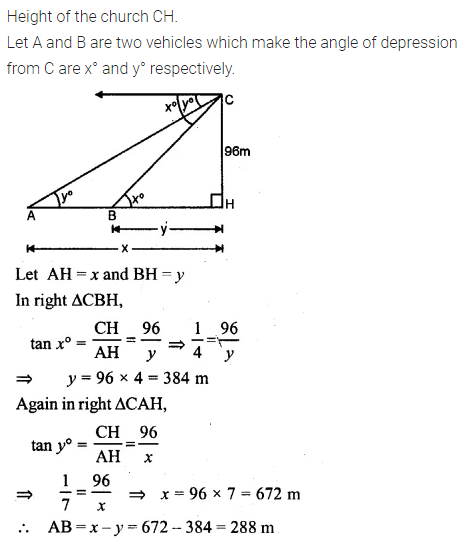Question 24.
In the adjoining figure, not drawn to the scale, AB is a tower and two objects C and D are located on the ground, on the same side of AB. When observed from the top A of the tower, their angles of depression are 45° and 60°. Find the distance between the two objects. If the height of the tower is 300 m. Give your answer to the nearest metre. (1998)Solution:Question 25.
The horizontal distance between the two towers is 140 m. The angle of elevation of the top of the first tower, when seen from the top of the second tower, is 30°. If the height of the second tower is 60 m, find the height of the first tower.
Solution: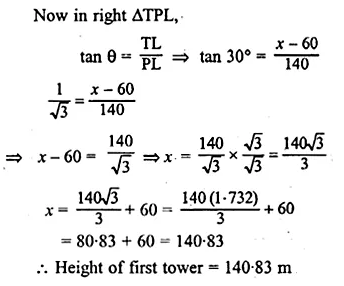Question 26.
As observed from the top of an 80 m tall lighthouse, the angles of depression of two ships on the same side of the lighthouse in a horizontal line with its base are 30° and 40° respectively. Find the distance between the two ships. Give your answer correct to the nearest metre.
Solution: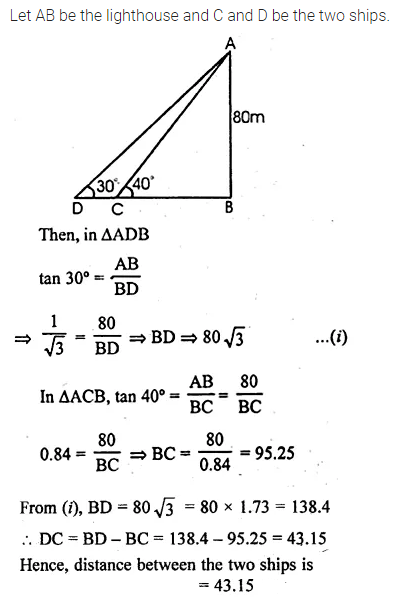Question 27.
The angle of elevation of a pillar from a point A on the ground is 45° and from a point B diametrically opposite to A and on the other side of the pillar is 60°. Find the height of the pillar, given that the distance between A and B is 15 m.
Solution: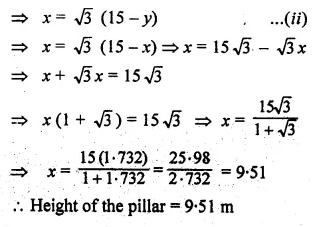Question 28.
From two points A and B on the same side of a building, the angles of elevation of the top of the building are 30° and 60° respectively. If the height of the building is 10 m, find the distance between A and B correct to two decimal places
Solution:Question 29.
(i) The angles of depression of two ships A and B as observed from the top of a lighthouse 60 m high are 60° and 45° respectively. If the; two ships are on the opposite sides of the lighthouse, find the distance between the two ships. Give your answer correct to the nearest whole number. (2017)
(ii) An aeroplane at an altitude of 250 m observes the angle of depression of two boats on the opposite banks of a river to be 45° and 60° respectively. Find the width of the river. Write the answer correct to the nearest whole number. (2014)
Solution: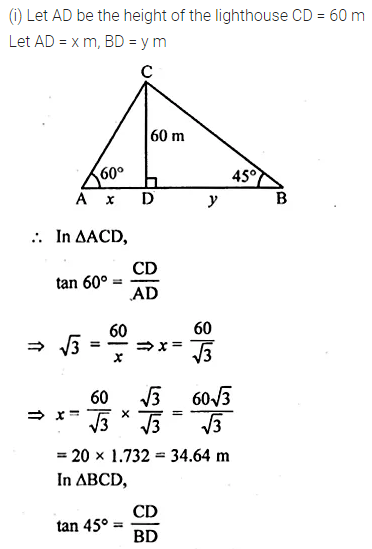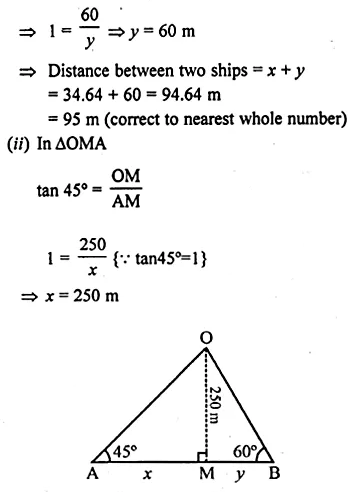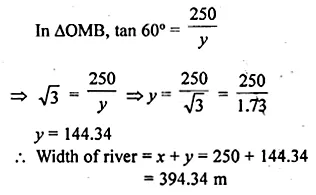Question 30.
From a tower, 126 m high, the angles of depression of two rocks which are in a horizontal line through the base of the tower are 16° and 12°20′ Find the distance between the rocks if they are on
(i) the same side of the tower
(ii) the opposite sides of the tower.
Solution: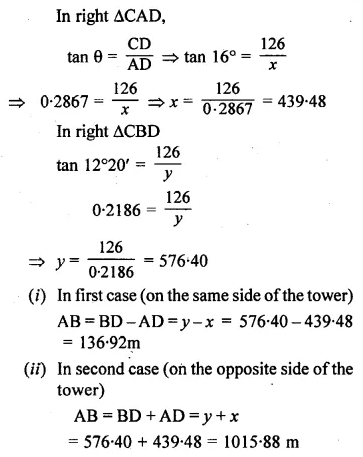Question 31.
A man 1.8 m high stands at a distance of 3.6 m from a lamp post and casts a shadow of 5.4 m on the ground. Find the height of the lamp post.
Solution: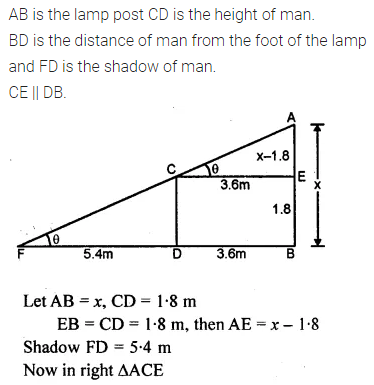Question 32.
The angles of depression of the top and the bottom of an 8 m tall building from the top of a multi-storeyed building are 30° and 45° respectively. Find the height of tire multi-storeyed building and the distance between the two buildings, correct to two decimal places.
Solution: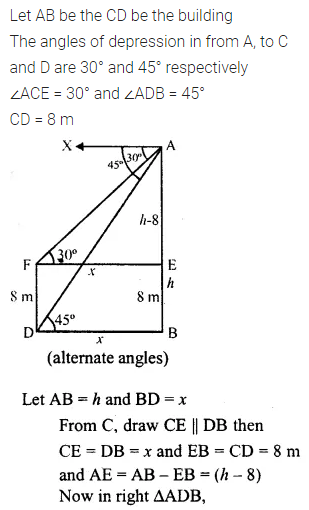Question 33.
A pole of height 5 m is fixed on the top of a tower. The angle of elevation of the top of the pole as observed from a point A on the ground is 60° and the angle of depression of the point A from the top of the tower is 45°. Find the height of the tower. (Take √3 = 1.732).
Solution:Question 34.
A vertical pole and a vertical tower are on the same level ground. From the top of the pole the angle of elevation of the top of the tower is 60° and the angle of depression of the foot of the tower is 30°. Find the height of the tower if the height of the pole is 20 m.
Solution: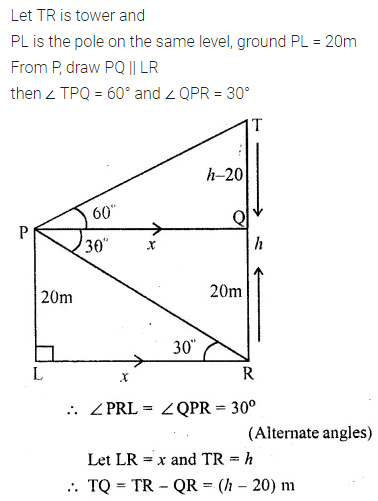Question 35.
From the top of a building 20 m high, the angle of elevation of the top of a monument is 45° and the angle of depression of its foot is 15°. Find the height of the monument.
Solution: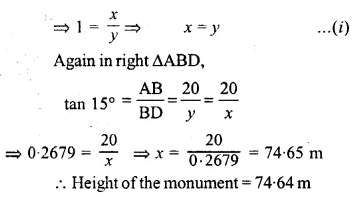Question 36.
The angle of elevation of the top of an unfinished tower at a point distant 120 m from its base is 45°. How much higher must the tower be raised so that its angle of elevation at the same point may be 60°?
Solution: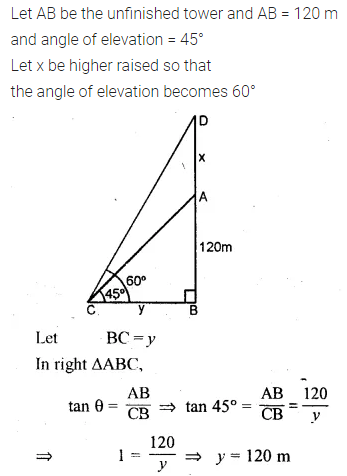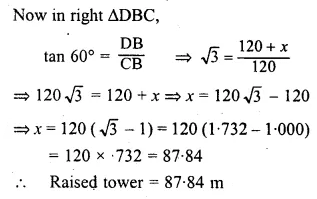Question 37.
In the adjoining figure, the shadow of a vertical tower on the level ground increases by 10 m, when the altitude of the sun changes from 45° to 30°. Find the height of the tower and give your answer, correct to $$\\ \frac { 1 }{ 10 }$$ of a metre.[Remark. The altitude of the sun means the angle of elevation of the sun.]
Solution: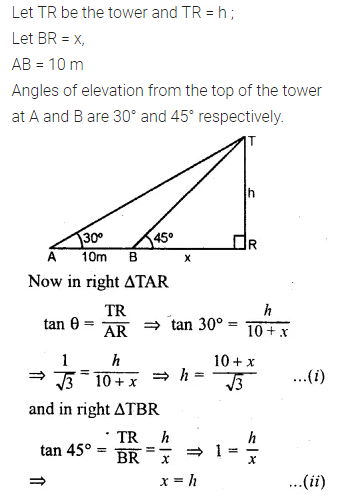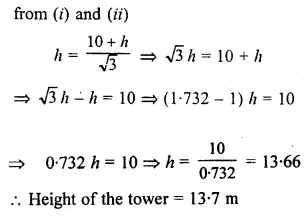Question 38.
An aircraft is flying at a constant height with a speed of 360 km/h. From a point on the ground, the angle of elevation of the aircraft at an instant was observed to be 45°. After 20 seconds, the angle of elevation was observed to be 30°. Determine the height at which the aircraft is flying (use √3 = 1.732)
Solution: